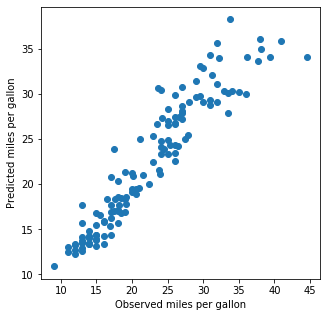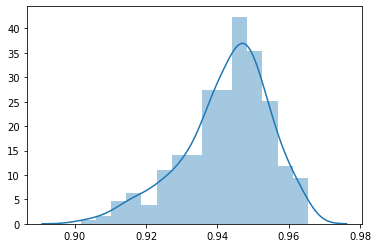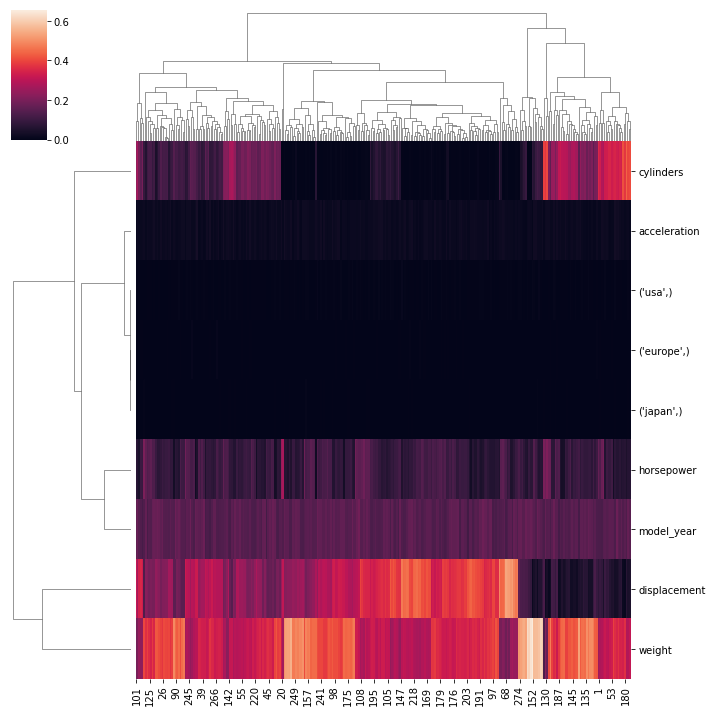# Predict Miles per Gallon

Last updated: April 27th, 2020

# 0. Initialize common libraries¶

In :
import numpy as np
import pandas as pd
import seaborn as sns

import matplotlib.pyplot as plt


In :
# Load example dataset (same as Bethany Baumann used for data visualization
# https://notebooks.ai/Beth1126/pda-python-dataframes-and-graphs-webinar-2020-54351877)

In :
data.head() # dataset contains information on cars

Out:
mpg cylinders displacement horsepower weight acceleration model_year origin name
0 18.0 8 307.0 130.0 3504 12.0 70 usa chevrolet chevelle malibu
1 15.0 8 350.0 165.0 3693 11.5 70 usa buick skylark 320
2 18.0 8 318.0 150.0 3436 11.0 70 usa plymouth satellite
3 16.0 8 304.0 150.0 3433 12.0 70 usa amc rebel sst
4 17.0 8 302.0 140.0 3449 10.5 70 usa ford torino

# 2. Data cleaning¶

## 2.1. Column to predict¶

In :
# Let us find the columns, which we want to predict and format it
feature_to_predict = 'mpg'  # miles per gallon


## 2.2.1. Numeric features¶

In :
ordinal_features = [
'cylinders',
'displacement',
'horsepower',
'weight',
'acceleration',
'model_year'
]

features_ordinal = data[ordinal_features].astype(float)

In :
if any(features_ordinal.isnull()):
print('At least some features have absent values')

At least some features have absent values

In :
# Make educated guesses on missing values (impute)
from sklearn.impute import SimpleImputer   # note that sklearn will soon get better ones
imputer = SimpleImputer(missing_values=np.nan, strategy='median')
imputed_values = imputer.fit_transform(features_ordinal.values)

In :
# For consistency, store data in original format (DataFrame)
features_ordinal = pd.DataFrame(
index=features_ordinal.index,
data=imputed_values,
columns=features_ordinal.columns
)


## 2.2.2. Nominal features¶

In :
nominal_features = [
'origin'
]

In :
from sklearn.preprocessing import OneHotEncoder
onehotencoder = OneHotEncoder()

one_hot_feature = onehotencoder.fit_transform(
data[nominal_features]).toarray()

In :
one_hot_feature

Out:
array([[0., 0., 1.],
[0., 0., 1.],
[0., 0., 1.],
...,
[0., 0., 1.],
[0., 0., 1.],
[0., 0., 1.]])
In :
onehotencoder.categories_

Out:
[array(['europe', 'japan', 'usa'], dtype=object)]
In :
features_nominal = pd.DataFrame(
index=data.index,
columns=onehotencoder.categories_,
data=one_hot_feature

)

In :
features_nominal.head(3)

Out:
europe japan usa
0 0.0 0.0 1.0
1 0.0 0.0 1.0
2 0.0 0.0 1.0

## 2.2.3. Combine ordinal and nominal features¶

In :
features = pd.concat(
[features_ordinal, features_nominal],
axis='columns'
)

In :
features.head()

Out:
cylinders displacement horsepower weight acceleration model_year (europe,) (japan,) (usa,)
0 8.0 307.0 130.0 3504.0 12.0 70.0 0.0 0.0 1.0
1 8.0 350.0 165.0 3693.0 11.5 70.0 0.0 0.0 1.0
2 8.0 318.0 150.0 3436.0 11.0 70.0 0.0 0.0 1.0
3 8.0 304.0 150.0 3433.0 12.0 70.0 0.0 0.0 1.0
4 8.0 302.0 140.0 3449.0 10.5 70.0 0.0 0.0 1.0

# 3. Machine Learning¶

## 3.1. Split training and testing set¶

In :
from sklearn.model_selection import train_test_split

In :
# per informal convention we will use
#  X to designate our features and
#  y as the value that should be predicted
X_train, X_test, y_train, y_test = train_test_split(
features,
test_size=0.33)

In [ ]:



## 3.2. Initialize a Gradient Boosting Model¶

Gradient Boosting will essentially perform two steps: First it will create a decision based model on the data. Second it will create a model of its own error. Together this means that 1) gradient boosting models can accomodate very distinct non-linear relationships between features and the entity to be predicted; 2) they are quite robust against being supplied too many (non-informative) features

In :
from sklearn import ensemble
gradient_booster.fit(X_train, y_train) # train with a subset of our data

/usr/local/lib/python3.8/site-packages/sklearn/ensemble/_gb.py:1454: DataConversionWarning: A column-vector y was passed when a 1d array was expected. Please change the shape of y to (n_samples, ), for example using ravel().
y = column_or_1d(y, warn=True)

Out:
GradientBoostingRegressor(alpha=0.9, ccp_alpha=0.0, criterion='friedman_mse',
init=None, learning_rate=0.1, loss='ls', max_depth=3,
max_features=None, max_leaf_nodes=None,
min_impurity_decrease=0.0, min_impurity_split=None,
min_samples_leaf=1, min_samples_split=2,
min_weight_fraction_leaf=0.0, n_estimators=100,
n_iter_no_change=None, presort='deprecated',
random_state=None, subsample=1.0, tol=0.0001,
validation_fraction=0.1, verbose=0, warm_start=False)

## 3.3. Test performance of our model¶

In :
y_predicted = gradient_booster.predict(X_test)

In :
plt.figure(figsize=(5,5))
plt.scatter(y_test, y_predicted)
plt.xlabel('Observed miles per gallon')
plt.ylabel('Predicted miles per gallon')

Out:
Text(0, 0.5, 'Predicted miles per gallon')In :
from scipy.stats import spearmanr

In :
spearmanr(y_test, y_predicted)

Out:
SpearmanrResult(correlation=0.9558187892787617, pvalue=5.498595266591838e-71)

## 3.4. Inspect models¶

To understand, which features drive the prediction.

In :
gradient_booster.feature_importances_

Out:
array([4.87671938e-03, 3.54394486e-01, 8.63099252e-02, 3.44312601e-01,
2.42777019e-02, 1.76290291e-01, 7.44785533e-04, 2.41898341e-04,
8.55159126e-03])
In :
features.head(2)

Out:
cylinders displacement horsepower weight acceleration model_year (europe,) (japan,) (usa,)
0 8.0 307.0 130.0 3504.0 12.0 70.0 0.0 0.0 1.0
1 8.0 350.0 165.0 3693.0 11.5 70.0 0.0 0.0 1.0
In :
pd.Series(
index=features.columns,

Out:
displacement    0.354394
weight          0.344313
model_year      0.176290
horsepower      0.086310
acceleration    0.024278
(usa,)          0.008552
cylinders       0.004877
(europe,)       0.000745
(japan,)        0.000242
dtype: float64

# (optional) 4. Iterate to test robustness¶

if running above multiple times, you will notice that some of the numbers (correlation between predicted and measured; and features importances) will change. This is largely because you will have different training and test sets.

## 4.1. Run multiple iterations, and gather statistics¶

In :
iterations = 300

In :
correlations = []

feature_importances = pd.DataFrame(
index=features.columns,
columns=range(iterations),
data=np.nan

)

In :
for j in range(iterations):

X_train, X_test, y_train, y_test = train_test_split(

correlations.append(spearmanr(y_test, y_predicted))


## 4.2. Inspect¶

In :
# Distribution of predicted correlations
sns.distplot(correlations)

Out:
<matplotlib.axes._subplots.AxesSubplot at 0x7fb48128eb80>In :
# Sanity check: are predictions better than any single correlation?
data.corr('spearman').loc['mpg', :]

Out:
mpg             1.000000
cylinders      -0.821864
displacement   -0.855692
horsepower     -0.853616
weight         -0.874947
acceleration    0.438677
model_year      0.573469
Name: mpg, dtype: float64
In :
# Feature importances
sns.clustermap(feature_importances)

Out:
<seaborn.matrix.ClusterGrid at 0x7fb4813c56a0>In [ ]:


In [ ]:


In [ ]:


In [ ]:


In [ ]:


In [ ]: